# How to Concatenate Column Values in Pandas DataFrame?

Many times we need to combine values in different columns into a single column. There can be many use cases of this, like combining first and last names of people in a list, combining day, month, and year into a single column of Date, etc. Now we’ll see how we can achieve this with the help of some examples.

Example 1: In this example, we’ll combine two columns of first name last name to a column name. To achieve this we’ll use the map function.

 `import` `pandas as pd ` `from` `pandas ``import` `DataFrame  ` `  `  `# creating a dictionary of names ` `Names ``=` `{``'FirstName'``:[``'Suzie'``,``'Emily'``,``'Mike'``,``'Robert'``], ` `         ``'LastName'``:[``'Bates'``,``'Edwards'``,``'Curry'``,``'Frost'``]} ` `  `  `# creating a dataframe from dictionary ` `df ``=` `DataFrame(Names, columns``=``[``'FirstName'``,``'LastName'``]) ` `print``(df) ` `  `  `print``(``'\n'``) ` `  `  `# concatenating the columns ` `df[``'Name'``] ``=` `df[``'FirstName'``].``map``(``str``) ``+` `' '` `+` `df[``'LastName'``].``map``(``str``) ` `print``(df) `

Output: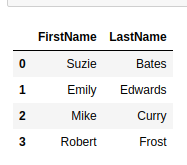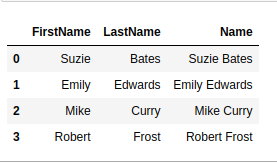Example 2: Similarly, we can concatenate any number of columns in a dataframe. Let’s see through another example to concatenate three different columns of the day, month, and year in a single column Date.

 `import` `pandas as pd ` `from` `pandas ``import` `DataFrame  ` ` `  `# creating a dictionary of Dates ` `Dates ``=` `{``'Day'``: [``1``, ``29``, ``23``, ``4``, ``15``],  ` `        ``'Month'``: [``'Aug'``, ``'Feb'``, ``'Aug'``, ``'Apr'``, ``'Mar'``],  ` `        ``'Year'``: [``1947``, ``1983``, ``2007``, ``2011``, ``2020``]} ` ` `  `# creating a dataframe from dictionary ` `df ``=` `DataFrame(Dates, columns ``=` `[``'Day'``, ``'Month'``, ``'Year'``]) ` `print` `(df) ` ` `  `print``(``'\n'``) ` ` `  `# concatenating the columns ` `df[``'Date'``] ``=` `df[``'Day'``].``map``(``str``) ``+` `'-'` `+` `df[``'Month'``].``map``(``str``) ``+` `'-'` `+` `df[``'Year'``].``map``(``str``) ` `print` `(df) `

Output: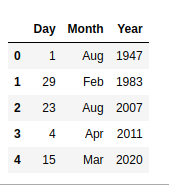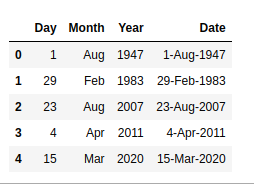Example 3:

We can take this process further and concatenate multiple columns from multiple different dataframes. In this example, we combine columns of dataframe df1 and df2 into a single dataframe.

 `import` `pandas as pd ` `from` `pandas ``import` `DataFrame  ` ` `  `# creating a dictionary of Dates ` `Dates ``=` `{``'Day'``: [``1``, ``1``, ``1``, ``1``],  ` `        ``'Month'``: [``'Jan'``, ``'Jan'``, ``'Jan'``, ``'Jan'``],  ` `        ``'Year'``: [``2017``, ``2018``, ``2019``, ``2020``]}  ` ` `  `# creating a dataframe from dictionary ` `df1 ``=` `DataFrame(Dates, columns ``=` `[``'Day'``, ``'Month'``, ``'Year'``])  ` ` `  `# creating a dictionary of Rates ` `Rates ``=` `{``'GDP'``: [``5.8``, ``7.6``, ``5.6``, ``4.1``],  ` `         ``'Inflation Rate'``: [``2.49``, ``4.85``, ``7.66``, ``6.08``]}  ` ` `  `# creating a dataframe from dictionary ` `df2 ``=` `DataFrame(Rates, columns ``=` `[``'GDP'``, ``'Inflation Rate'``]) ` ` `  `# combining columns of df1 and df2 ` `df_combined ``=` `df1[``'Day'``].``map``(``str``) ``+` `'-'` `+` `df1[``'Month'``].``map``(``str``) ``+` `'-'` `+` `df1[``'Year'``].``map``(``str``) ``+` `': '` `+` `'GDP: '` `+` `df2[``'GDP'``].``map``(``str``) ``+` `'; '` `+` `'Inflation: '` `+` `df2[``'Inflation Rate'``].``map``(``str``) ` `print` `(df_combined) `

Output: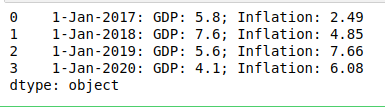My Personal Notes arrow_drop_upCheck out this Author's contributed articles.

If you like GeeksforGeeks and would like to contribute, you can also write an article using contribute.geeksforgeeks.org or mail your article to contribute@geeksforgeeks.org. See your article appearing on the GeeksforGeeks main page and help other Geeks.

Please Improve this article if you find anything incorrect by clicking on the "Improve Article" button below.

Article Tags :

Be the First to upvote.

Please write to us at contribute@geeksforgeeks.org to report any issue with the above content.